# C++ Pointer: The Complete Guide

To understand the pointer, let’s review the “regular” variables first. If you’re familiar with the programming language without pointers like JavaScript, this is what you think when you hear “variable”.

## C++ Pointer

C++ pointer is a variable whose value is the address of another variable. Pointers are a symbolic representation of addresses. Pointers enable programs to simulate call-by-reference and create and manipulate dynamic data structures.

You must declare the pointer before you can work with it. When declaring a variable by an identifier (or name), the variable is synonymous with its value.

```int number = 3;
std::cout << "number is initialized with a value of " << number << "\n";
// Outputs: number is initialized with a value of 3```

The value of a variable can be modified directly.

```number++;
number = number * 2;
std::cout << "After modifying number, its value is now " << number << "\n";
// Outputs: After modifying number, its value is now 8```

Every value lives at memory addresses. The memory address of a variable can be accessed using the & operator.

`std::cout << "The number variable's value lives at memory address " << &number << "\n";`

The address of the variable can be obtained by preceding the name of a variable with an ampersand sign (&), known as an address-of operator. See the following code.

`app = &var;`

This would assign the address of a variable var to an app; by preceding the name of the variable var with the address-of operator (&), we are no longer assigning the content of the variable itself to an app but its address.

The actual address of the variable in memory cannot be known before runtime, but let’s assume, to help clarify some concepts, that var is placed during runtime in the memory address 1921.

## What is Pointer?

Pointers are different entities compared to standard variables because they store the address of some other variable or a waypoint to a variable.

Ex.  int a; //’a’ is a variable that will hold some integer value.

int *a; // ‘a’ is a pointer to a integer variable.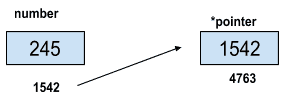### General Declaration

```<type> * pointerName;    <type> can be int, float, char, etc.
```

## Why use C++ pointers?

As mentioned earlier, pointers hold the address, so we can use it to reduce complexity while sending parameters in the function.

Suppose there is an array of enormous size, and we have to send the array as a parameter of the function, so instead of passing the array, we can give the address of the first variable.

Also, computing operations using pointers at the address level are less complicated than those without them.

Sample Example:

To know the address of a variable, we use the referencing operator ‘&’, and to see the value stored at the address pointed by a pointer, we use dereferencing operator ‘*’.

```int a = 10;  // integer variable a
int *p = &a;  // referencing operator ‘&’ prefixed with integer variable a. p will now store the address of a.
int b = *p; // dereferencing pointer p using ‘*’ prefixed with p. b will store the value of a that is 10.
```

See the following pointer program in C++.

```#include <iostream>
using namespace std;

int main()
{
// Sample program to show pointers concepts.
int a=20; // integer variable a
int * p; // pointer to an integer
p = &a;  // p now holds address of a.

cout << "Address of a : " << p << "\n";

int b = *p;  // dereferencing using *, now b has the same value as a.
cout << "Value of a: " << *p << "\n";

return 0;
}
```

See the output.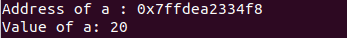### Applications:

Used in calling by reference using pointers.

When a variable is passed as a reference in call-by reference, changes made to it inside the function body are also reflected in the main function. For example, consider the below program of swapping two numbers using pointers.

```#include <iostream>
using namespace std;

void swap_(int *x, int *y)
{
int temp= *x; // store the value stored at address pointed by x.
*x = *y;      // change value at address pointed by x to value at address y
*y = temp;   // change value at address pointed by y to value that was present at address of x at start.
}
int main()
{
// Sample program to show call by reference by pointers
int a=20; // integer variable a
int b=30; // integer variable b
cout << "Before swapping : " << a << " " << b << "\n";
swap_(&a,&b); // swap function

cout << "After swapping : " << a << " " << b << "\n";
return 0;
}
```

After the swap function is called, values are swapped between a & b, a=30 and b=20. See the output.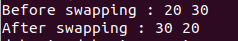## C++ Array of Pointers

An array of pointers in C++ means an array that stores addresses of a different variable.

`int * a;  // array of integer pointers`

See the following code example.

```#include <iostream>
using namespace std;

int main()
{
// Sample program to show call by reference by pointers
int a;
int i,j;
for(i=0;i<10;i++)
a[i]=i+1;
cout << "Without pointers :\n";
for(i=0;i<10;i++)
cout << a[i] << " ";
cout << "\n";
int *p; // pointers to each member of array of a

for(i=0;i<10;i++)
p[i] = &a[i];

cout << "Using pointers :\n";
for(i=0;i<10;i++)
cout << *p[i] << " ";

return 0;
}
```

See the output.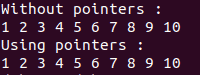## Pointers and Array

The name of the array is the starting address of the array. So, consider int a; a , &a , &a signify the same thing.

To access ith index in the array it can be done using *(a+i), *(i+a) , a[i] or i[a]. All are the same.

See the following code example.

```#include <iostream>
using namespace std;

int main()
{
// Sample program to show call by reference by pointers
int a;
int i,j;
for(i=0;i<10;i++)
a[i]=i+1;
//All point to the same address
cout << a << " " << &a << " " << &a << "\n" ;
// All give the same value that is the value contained at a
cout << *(a+1) << " " << *(1+a) << " " << a << " " << 1[a] <<"\n";

return 0;
}
```

See the following output.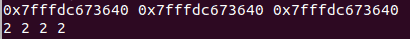## Pointers Arithmetic

Arithmetic operations like ++, –, + , – can be operated on pointer variables.

Consider, int a = {1,2,3};

```ptr = &a;

ptr = ptr+1; // This will point to the next variable in the array. ptr++ will do the same thing.

Ptr = ptr-1; // Again the pointer will point to the 0th index. ptr—will do the same thing.```

See the following code.

```#include <iostream>
using namespace std;

int main()
{
// Sample program to show call by reference by pointers
int a = {1,2,3};
int * p= &a;
p=p+1; // point to next integer

cout << "Value of 2nd element : " << *p << "\n";

p--;
cout << "Value of 1st element : " << *p << "\n";

return 0;
}
```

See the output.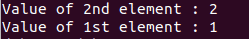Some essential types of pointers are the following.

### Null pointer in C++

A NULL pointer in C++  points to nothing and has been initialized to NULL. A NULL pointer is a 0 value.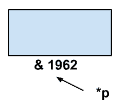See the following code.

```#include <iostream>
using namespace std;

int main()
{
// Sample program to show Null pointer
int * p = NULL; // initialized to NULL

cout << "VALUE : " << p << "\n";

return 0;
}
```

See the output.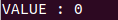### Void Pointer in C++

Void is a type of pointer; unlike the NULL pointer. Typecasting needs to be used while dealing with void pointers. Using this pointer, you can efficiently work with any data type. You need to include that data type.

See the following program.

```#include <iostream>
using namespace std;

int main()
{
// Sample program to show Void pointer
int a=10;
void * p; // initialized to NULL

p = &a;  // address of a

cout << "VALUE : " << *((int*)p) << "\n"; // typecasting used otherwise there will be error.

return 0;
}
```

See the output.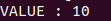## Dangling pointer

A pointer that points to a memory location that no longer exists, that is, the memory location has been deleted. See the following program.

```#include <iostream>
using namespace std;

int main()
{
// Sample program to show dangling pointer
int *a = new int;
a = 10;
a = 20;
int *p = &a;
delete a;    // comment this line to avoid dangling pointer

cout <<"Value is: "<<*p<<"\n";  // Garbage value will be printed
return 0;
}
```

See the following output.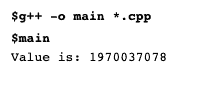## C++ Pointer to pointer

A pointer that stores the address of another pointer is called a pointer to a pointer. Here, in this case, a pointer stores the address of another pointer, and then that 2nd pointer stores the address of one variable.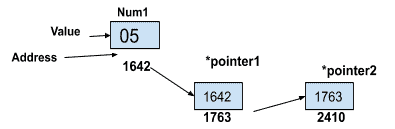The following program tells how to deal with it.

```#include <iostream>
using namespace std;

int main()
{
// Sample program to show  pointer to pointer
int a = 10;
int *p = &a; // stores the address of integer a
int **q = &p; // stores the address of pointer p

cout << "Address of a : " << p << "\n";
cout << "Address of p : " << q << "\n";

cout << "Value of p : " << p << " " << *q << "\n" ; // All are same

cout << "Value of a : " << a << " " << *p << " " << **q << "\n"; // All are same

return 0;
}
```

See the output.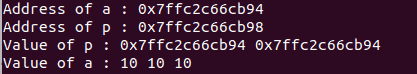That’s it.

Categories C++

This site uses Akismet to reduce spam. Learn how your comment data is processed.